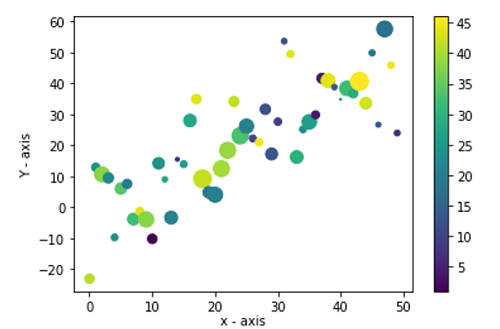# Python | Color Bar using Matplotlib

In this tutorial, we are going to learn how to add a Color Bar in a figure using Matplotlib?
Submitted by Anuj Singh, on August 05, 2020

It is helpful to use color bars augmented to a plot for better visualization and matplotlib have an inbuilt function for our desired operation. The following example shows us how to implement color bar in python using matplotlib.

Illustration:## Python code for color bar using matplotlib

```import numpy as np
import matplotlib.pyplot as plt

data = {'a': np.arange(50),
'c': np.random.randint(0, 50, 50),
'd': np.random.randn(50)}
data['b'] = data['a'] + 10 * np.random.randn(50)
data['d'] = np.abs(data['d']) * 100

plt.scatter('a', 'b', c='c', s='d', data=data)
plt.ylabel('Y - axis')
plt.colorbar()
plt.show()
```

Output:

```Output is as figure
```